### Forces and Laws of Motion - Solutions

CBSE class IX Science

NCERT Solutions
Chapter 9
Forces and Laws of Motion

(Page No. 118)

1. Which of the following has more inertia:

(a) a rubber ball and a stone of the same size?
(b) a bicycle and a train?
(c) a five rupees coin and a one-rupee coin?

Ans. (a) a stone of the same size will have more inertia than a rubber ball.
(b) A train will have more inertia than a bicycle.
(c) A five rupees coin will have more inertia than a one-rupee coin.

2. In the following example, try to identify the number of times the velocity of the ball changes:

“A football player kicks a football to another player of his team who kicks the football towards the goal. The goalkeeper of the opposite team collects the football and kicks it towards a player of his own team”.

Also, identify the agent supplying the force in each case.

Ans. In the given example the velocity of football changes four times. As described below:
(i) when the football player is supplying the force when he kicks the football to another player.
(ii) when the other player kicks football towards the goal.
(iii) When the goalkeeper of other team stops the ball.
(iv) When the goalkeeper kicks the football towards a player of his team.

3. Explain why some of the leaves may get detached from a tree if we vigorously shake its branch.

Ans. Some of the leaves may get detached from a tree if we vigorously shake its branch because the some of the leaves, due to the property of inertia remain at rest while we vigorously shake a branch of the tree, as a result, those leaves detach and fall off.

4. Why do you fall in the forward direction when a moving bus brakes to a stop and fall backward when it accelerates from rest?

Ans. when a moving bus brakes to a stop we fall in the forward direction because we are also moving with the speed of bus due to the inertia of motion and when suddenly it puts brakes i.e. comes to rest the lower half of our body also comes to rest but the upper half of our body not being in close contact with bus is still in the phase of motion so we fall in the forward direction.
When the bus accelerates from rest, we are also at rest being on the resting seat as the engine applies force in the forward direction we fall backward because lower part direct in touch with the bus but the upper part which is still in the inertia of rest because it is not in direct touch with the bus.

(Page No.126)

1. If action is always equal to the reaction, explain how a horse can pull a cart.

Ans. With a balancing force the overall impact is the absence of movement but with unbalanced forces, the resultant or the bigger force causes the motion. Same is true in the case where a horse pulls a cart. Horse exerts more force on the cart than the cart exerts to resist its movement hence this is an unbalanced force and the cart moves in the direction of horse’s pull.

2. Explain, why is it difficult for a fireman to hold a hose, which ejects large amounts of water at a high velocity.

Ans. It is difficult for a fireman to hold a hose, which ejects large amounts of water at a high velocity because of Newton's third law of motion; when the hose ejects large amounts of water at a high velocity in forwarding direction, the water coming out pushes the hose pipe in backward direction and it becomes difficult to hold it.

3. From a rifle of mass 4 kg, a bullet of mass 50 g is fired with an initial velocity of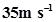. Calculate the initial recoil velocity of the rifle.

Ans. Initial Momentum of rifle and bullet (before fire)
= mv = (4kg + 0.05kg) x 0m/s = 0
Final Momentum of rifle and bullet (after fire)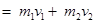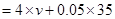where (50 g = 0.05 kg)

$v=\frac{-\left(0.05×35\right)}{4}$= - 0.44 m/s

4. Two objects of masses 100 g and 200 g are moving along the same line and direction with velocities of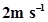and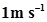, respectively. They collide and after the collision, the first object moves at a velocity of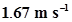. Determine the velocity of the second object.

Ans. Given,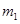= 100g = 0.1 kg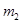= 200g = 0.2 kg

velocity of the first object =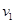= 2 m/s
velocity of the second object =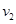= 1 m/s
Momentum before the collision =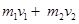= 0.1 x 2 + 0.2 x 1
= 0.4 kg m/s

since velocity of first object after collision = 1.67m/s
If we suppose that velocity of second object = v
As per law of conservation of momentum,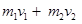(initial momentum = final momentum)
0.4 = 0.1 x 1.67 + 0.2 x v
0.4 = 0.167 + 0.2v
0.2v = 0.4 -0.167
v = 0.2333/0.2 = 2.33/2 = 1.16 m/s

(Chapter – end)

1. An object experiences a net zero external unbalanced force. Is it possible for the object to be travelling with a non-zero velocity? If yes, state the conditions that must be placed on the magnitude and direction of the velocity. If no, provide a reason.

Ans. No, it is not possible for the object to be travelling with a non-zero velocity if an object experiences a net zero external unbalanced force.According to Newton's first law of motion, a body continues to be in its state of rest or motion, unless it is acted upon by some unbalanced external force

2. When a carpet is beaten with a stick, dust comes out of it. Explain.

Ans. When a carpet is beaten with a stick, dust comes out of it because carpet fibers vibrate in forward and backward direction as the carpet is beaten but the loosely bound dust particles due to inertia remain at rest and so they come out.

3. Why is it advised to tie any luggage kept on the roof of a bus with a rope?

Ans. It is advised to tie any luggage kept on the roof of a bus with a rope because when bus moves the luggage also gets moving with the velocity same as that of the bus and in the same direction but when bus changes direction or deaccelerates, due to inertia of motion luggage moves in the same direction and because it is loosely attached to the bus and may get thrown away from roof of buses.

4. A batsman hits a cricket ball which then rolls on a level ground. After covering a short distance, the ball comes to rest. The ball slows to a stop because

(a) the batsman did not hit the ball hard enough.
(b) velocity is proportional to the force exerted on the ball.
(c) there is a force on the ball opposing the motion.
(d) there is no unbalanced force on the ball, so the ball would want to come to rest.

Ans. (c) there is a force on the ball opposing the motion.

5. A truck starts from rest and rolls down a hill with a constant acceleration. It travels a distance of 400 m in 20 s. Find its acceleration. Find the force acting on it if its mass is 7 metric tons (Hint: 1 metric ton = 1000 kg.)

Ans. According to the question,
initial velocity of truck (u) = 0
distance = s= 400 m and time = 20 s
mass of truck = 7metric tons = 7000kg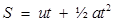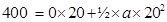400 =0 + 200a
400 = 200a
a = 400/200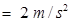therefore,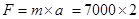= 14000 N

6. A stone of 1 kg is thrown with a velocity of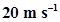across the frozen surface of a lake and comes to rest after travelling a distance of 50 m. What is the force of friction between the stone and the ice?

Ans. since v2 = u2 + 2as
0 = 202 + 2 x a x 50 (object comes to rest so v=0)
-100a = 400
a = 400/-100 = - 4m / sec2

therefore, the force of friction between the stone and the ice

$F=m×a$$=1×\left(-4m/{\mathrm{sec}}^{2}\right)$ = -4 N

7. An 8000 kg engine pulls a train of 5 wagons, each of 2000 kg, along a horizontal track. If the engine exerts a force of 40000 N and the track offers a friction force of 5000 N, then calculate:

(a) the net accelerating force
(b) the acceleration of the train; and
(c) the force of wagon 1 on wagon 2.

Ans. (a) The net accelerating force = Force exerted by engine - frictional force of track = 40000 - 5000 = 35000 N

(b) the acceleration of the train = a = F/m =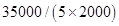= 35000/10000 =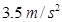(c) the force of wagon 1 on wagon 2
Wagon 1 will have to exert force on all 4 wagons next to it
so mass of other 4 wagons =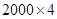= 8000 kg
F = ma =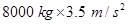= 28000 N

8. An automobile vehicle has a mass of 1500 kg. What must be the force between the vehicle and road if the vehicle is to be stopped with a negative acceleration of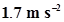?

Ans. since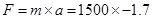= -2550 N (negative sign symbolises acceleration in opposite direction)

9. What is the momentum of an object of mass m, moving with a velocity v?

(a)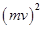(b)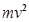(c)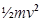(d) mv

Ans. (d) mv

10. Using a horizontal force of 200 N, we intend to move a wooden cabinet across a floor at a constant velocity. What is the friction force that will be exerted on the cabinet?

Ans. 200 N